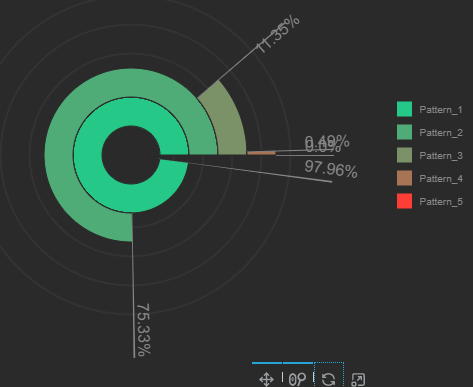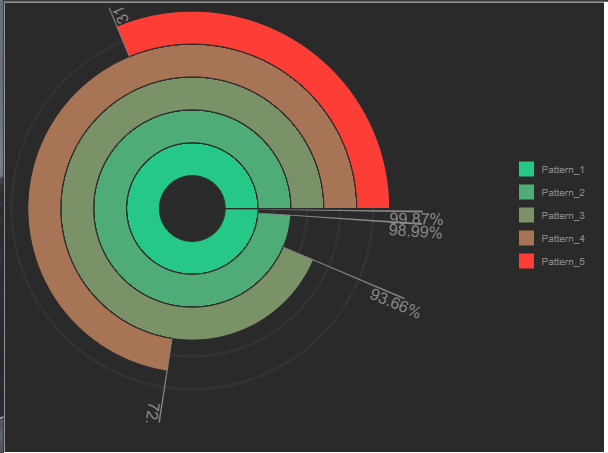# Texts in Charts

Hello all,

I tried to draw something like this in bokeh:

→ there is no problem to use “annular_wedge”, but problem is to determine correct position (x, y) and angle for text. I found is almost impossible to align it with the line:→ this is my result as you can see the text is always in different possition agains cetral point [0,0]

So to make it easier (not need to reproduce all what I have → SQL reads, .json reading …) I prepared one simple example to demonstrate issue:

Result:

→ Issue is that somehow when I use the text with angle (or without I dont know) the also distance from center differs.
→ the most evidence it is on x-axis where texts are at the circle and correclty attached to line, on other hand on y-axis they are a little bit stretch out from central point
→ also noticed that durind Zooming the text changes coordinates agains the rest of objects.

here is complete code I used:

``````from bokeh.plotting import figure, show
from math import pi, sin, cos

return x

return y

def round_percentage(item):
item = round(float(item),2)
item = str(f"{item}%")
return item

plot = figure(
title=None, width=500, height=500, x_range = [-4, 4], y_range = [-4, 4],
min_border=0)

# Main circle
plot.annular_wedge(
x = 0,
y = 0,
direction = "anticlock",
start_angle = 0,
end_angle = 2 * pi,
line_color = "black",
fill_color = "black")

N = 12
Angle_ratio = 2 * pi / N
Pre_angle = 0
for i in range(N):
New_angle = Pre_angle + Angle_ratio
Pre_angle = New_angle

anchor = ["top_left"] * N

# small steps
Pre_angle = 0
for i in range(N):
New_angle = Pre_angle + Angle_ratio
plot.annular_wedge(
x = 0,
y = 0,
direction = "anticlock",
start_angle = New_angle,
end_angle = New_angle + 0.01,
line_color = "black",
fill_color = "black")
Pre_angle = New_angle

plot.text(x=x, y=y, text=text, angle=angle, angle_units = "rad", text_color="#96deb3", anchor = anchor)

show(plot)
``````

My Bokeh Version is: 3.1
Python: 3.11.2
Using Windows 10
and displaying result in Microsoft Edge

thank you

Jan

For something like this you will definitely need to set `match_aspect=True` on the plot and also use auto-ranging rather than setting explicit ranges.

Hello Bryan,

thnak you forl hint, it is working correctly now, to text is correctl pointed to he x, y space. Unfortuantelly this coused that charts are a little bit zoomed in so not showing all data (especially I thing that is not counting with text lenght).

Another thing which I would like to understand is why the text is changing its size during zooming (is this because of units?)

Otherwise I really appreciate your help at all :-).

Here are results how my charts looks like right after generation (zoomedin and center is a little bit left):→ similar thing happened for code example (there is just a little bit zoomed in)

``````from bokeh.plotting import figure, show
from math import pi, sin, cos

return x

return y

def round_percentage(item):
item = round(float(item),2)
item = str(f"{item}%")
return item

plot = figure(
title=None, width=500, height=500,
min_border=0, match_aspect=True)

# Main circle
plot.annular_wedge(
x = 0,
y = 0,
direction = "anticlock",
start_angle = 0,
end_angle = 2 * pi,
line_color = "black",
fill_color = "black")

N = 12
Angle_ratio = 2 * pi / N
Pre_angle = 0
for i in range(N):
New_angle = Pre_angle + Angle_ratio
Pre_angle = New_angle

anchor = ["top_left"] * N

# small steps
Pre_angle = 0
for i in range(N):
New_angle = Pre_angle + Angle_ratio
plot.annular_wedge(
x = 0,
y = 0,
direction = "anticlock",
start_angle = New_angle,
end_angle = New_angle + 0.01,
line_color = "black",
fill_color = "black")
Pre_angle = New_angle

plot.text(x=x, y=y, text=text, angle=angle, angle_units = "rad", text_color="#96deb3", anchor = anchor)

show(plot)
``````

Thank you

Jan

I think having text participate more fully in auto-ranging is still a work in progress. cc @mateusz For the time being, if you need to “expand” things, a workaround would be to plot some invisible (alpha=0) circles at the corners of a “bounding box” you want to define.

Another thing which I would like to understand is why the text is changing its size during zooming (is this because of units?)

I am actually not sure I understand this one. We actually have several issues about the converse, i.e. the text not changing size with zoom, and that is what I would expect to see. I will have to actually run the code soon to investigate.

Bello

So ai tested first part,

For the time being, if you need to “expand” things, a workaround would be to plot some invisible (alpha=0) circles at the corners of a “bounding box” you want to define.

and circle was not working ( draw circle with higer radius, but zoom was still there). Only wgat helps was to draw 4 text on x and y axes (0°, 90° 180° and 270°), text was simple “.” and byt this it was working. Seems that this zoom efect is influenced by figure.text glym(parameter - anchor)

Solution:

``````# Helper invisible text to make Chart in center
N = 4
Pre_angle = 0
Angle_ratio = 2 * pi / N
text = ["."] * N
for i in range(N):
New_angle = Pre_angle + Angle_ratio